# Inter maths solutions for De Moivre’s Theorem

Intermediate mathematics textbook chapter 2 exercises 2(a) and 2(b) solutions are given.

These solutions are very easy to understand. First you study the textbook lesson.

Then observe the example problems and solutions. Try them in your own way.

Observe the solutions given below and try them in your own method.

You can also see

Inter Maths 1A textbook solutions

Inter Maths 1B textbook solutions

Inter Maths IIA textbook solutions

Inter Maths IIB textbook solutions

De Moivre’s theorem

M

## Inter maths solutions for De Moivre’s Theorem (textbook)

Chapter – 2 De Moivre’s Theorem

Exercise 2(a)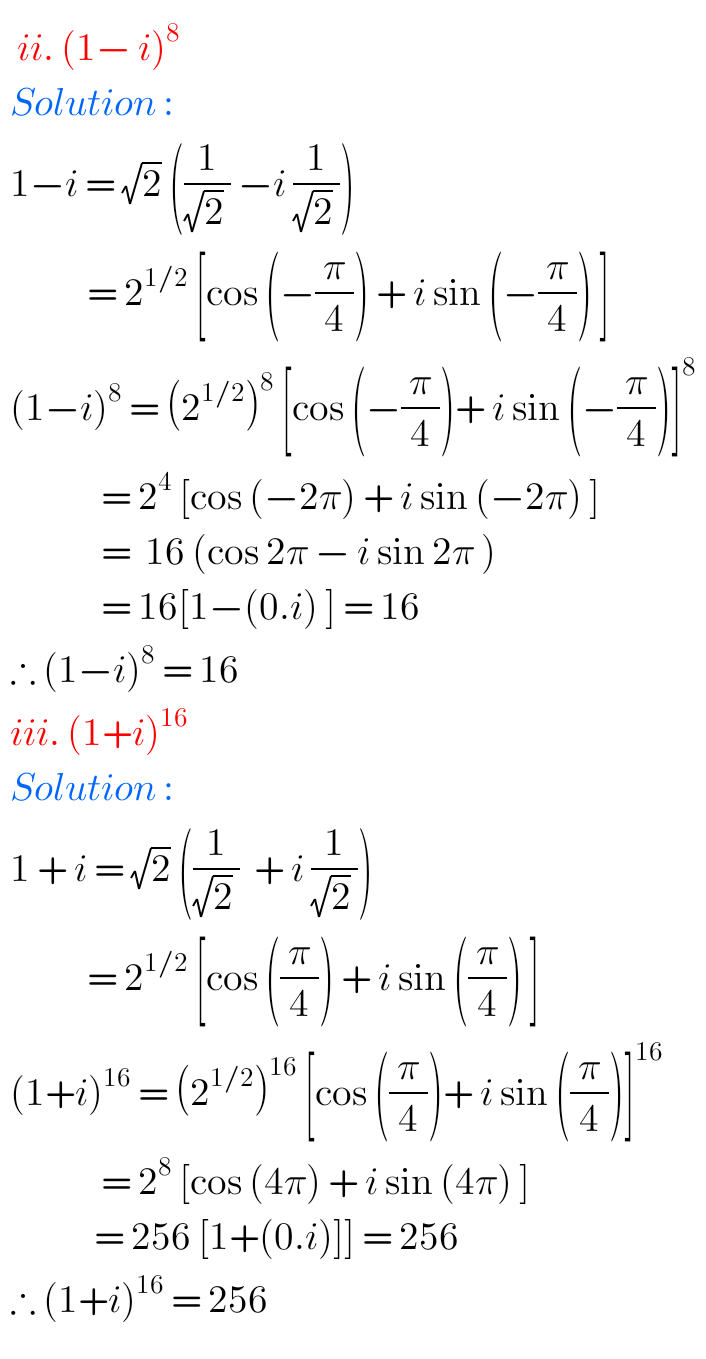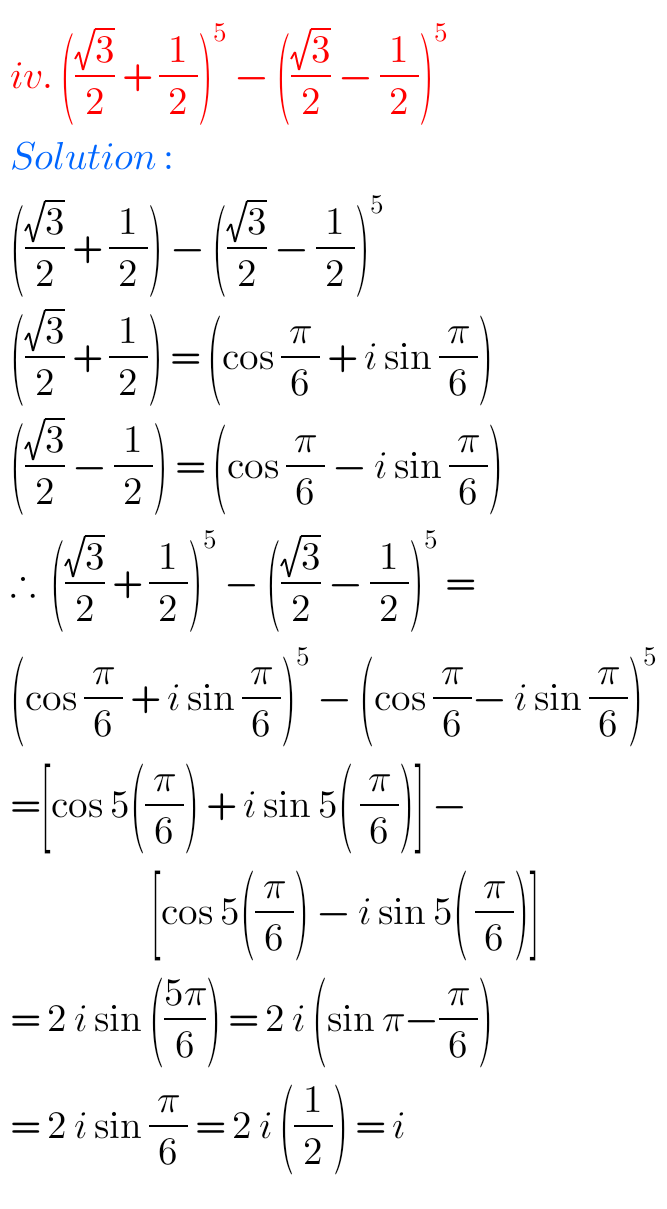###### Solutions for De Moivre’s Theorem Inter 2a maths

Exercise 2(b)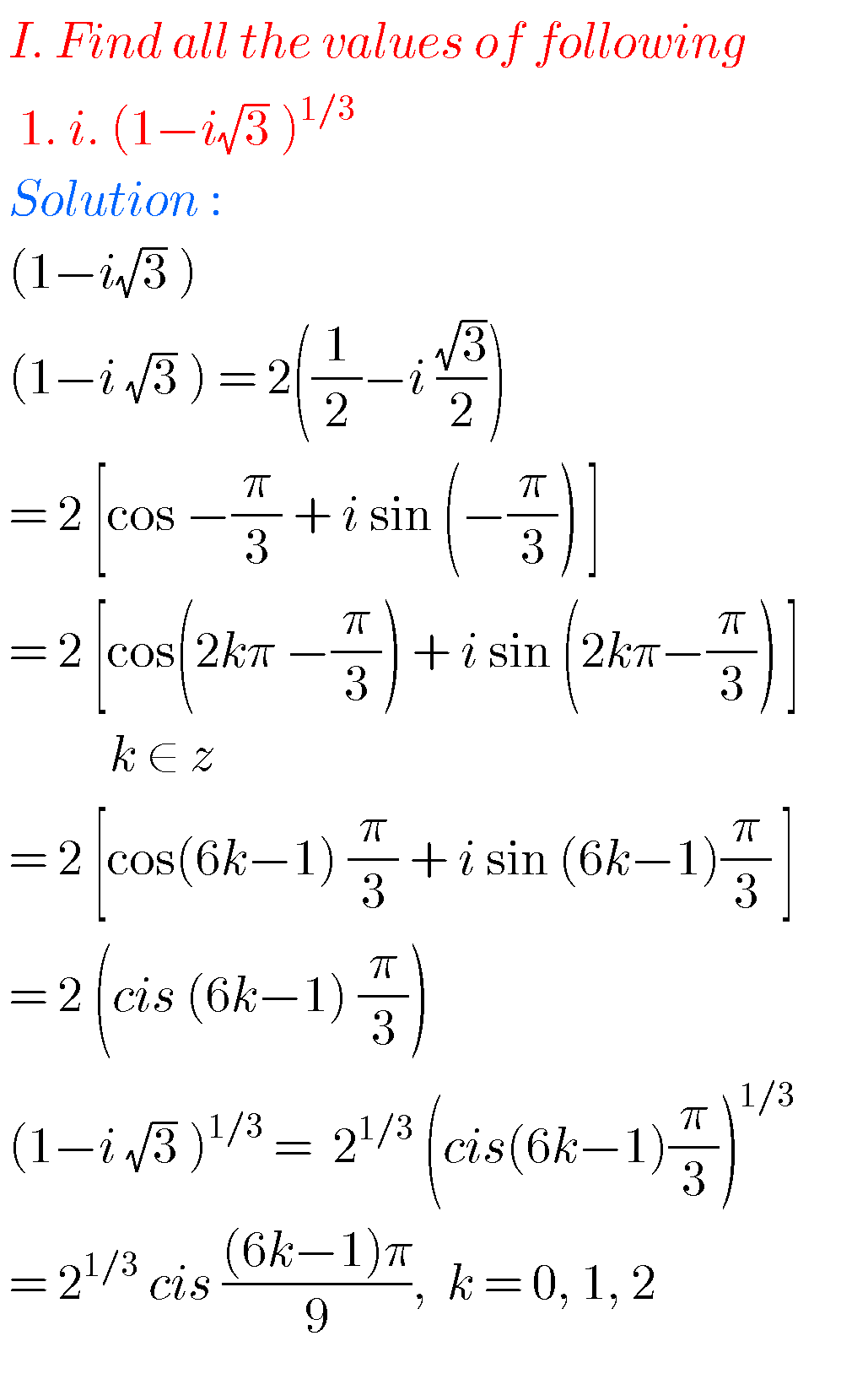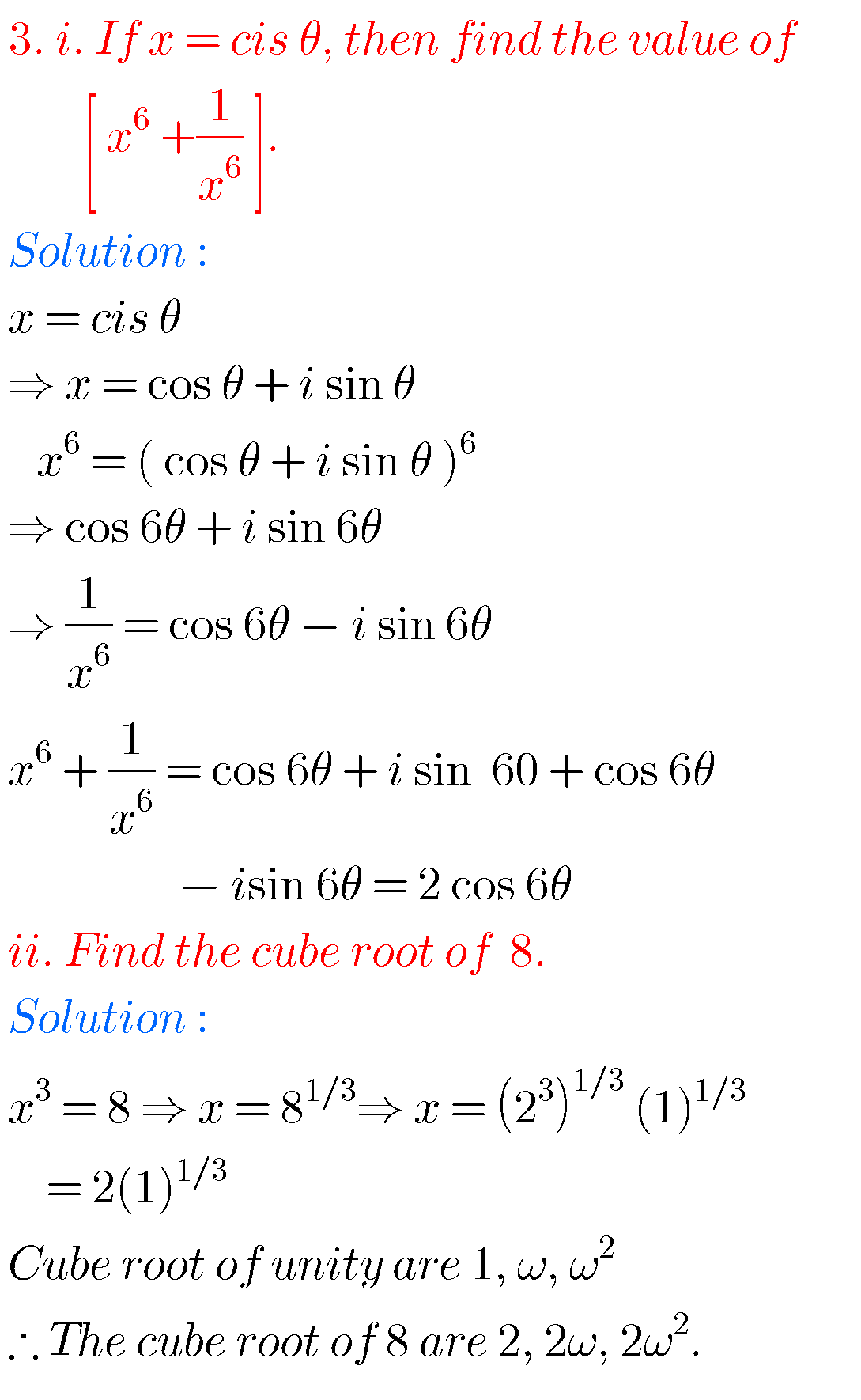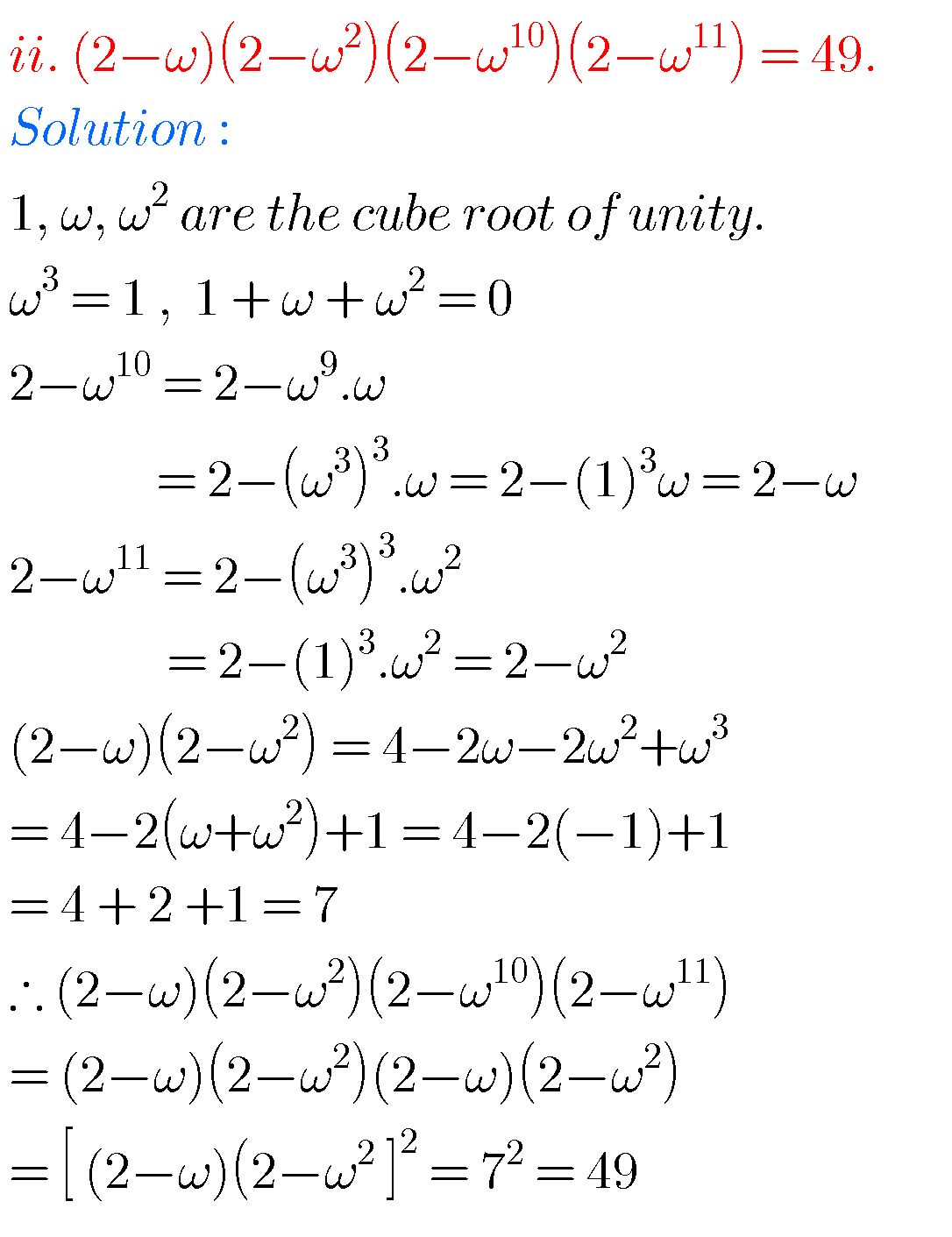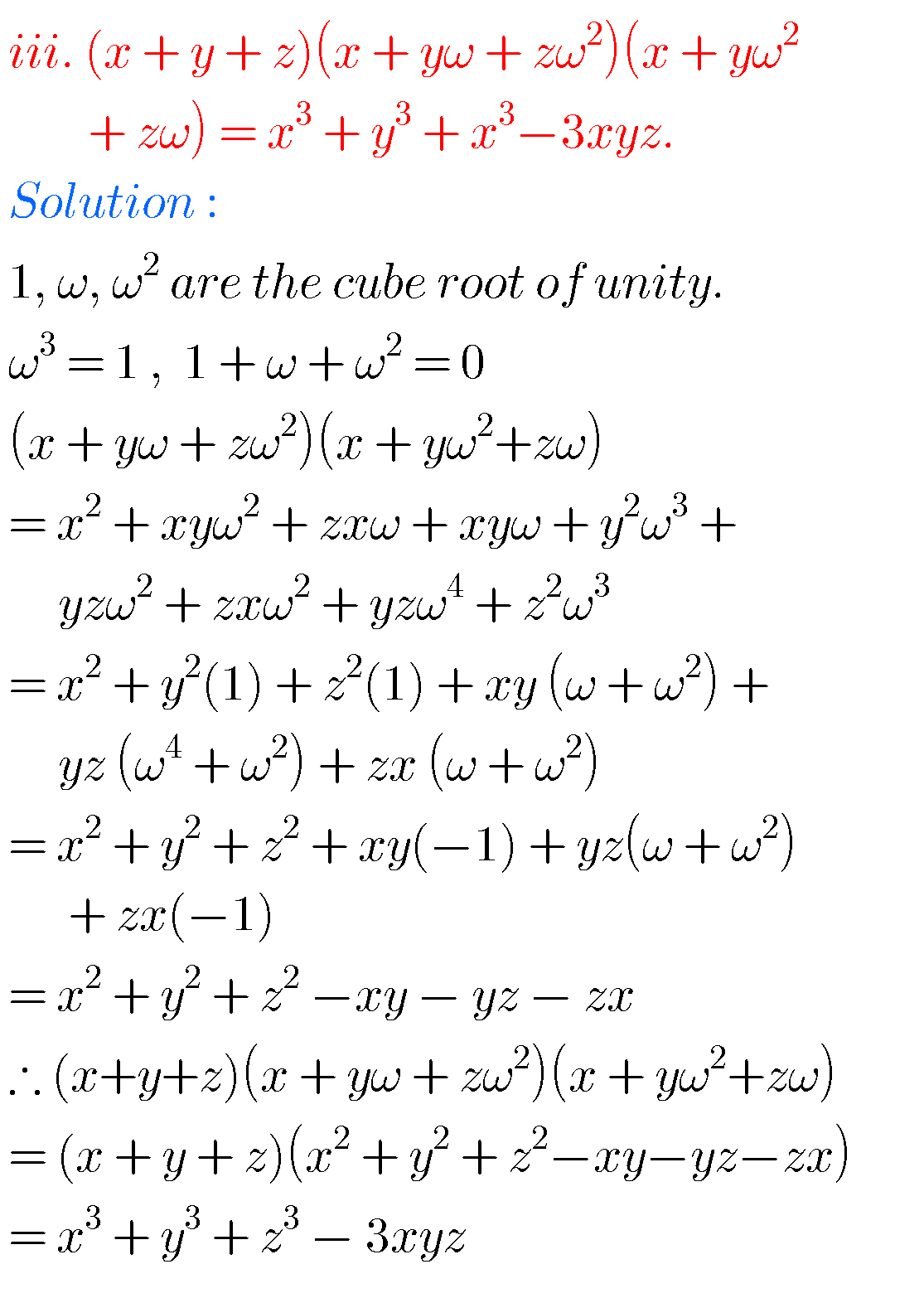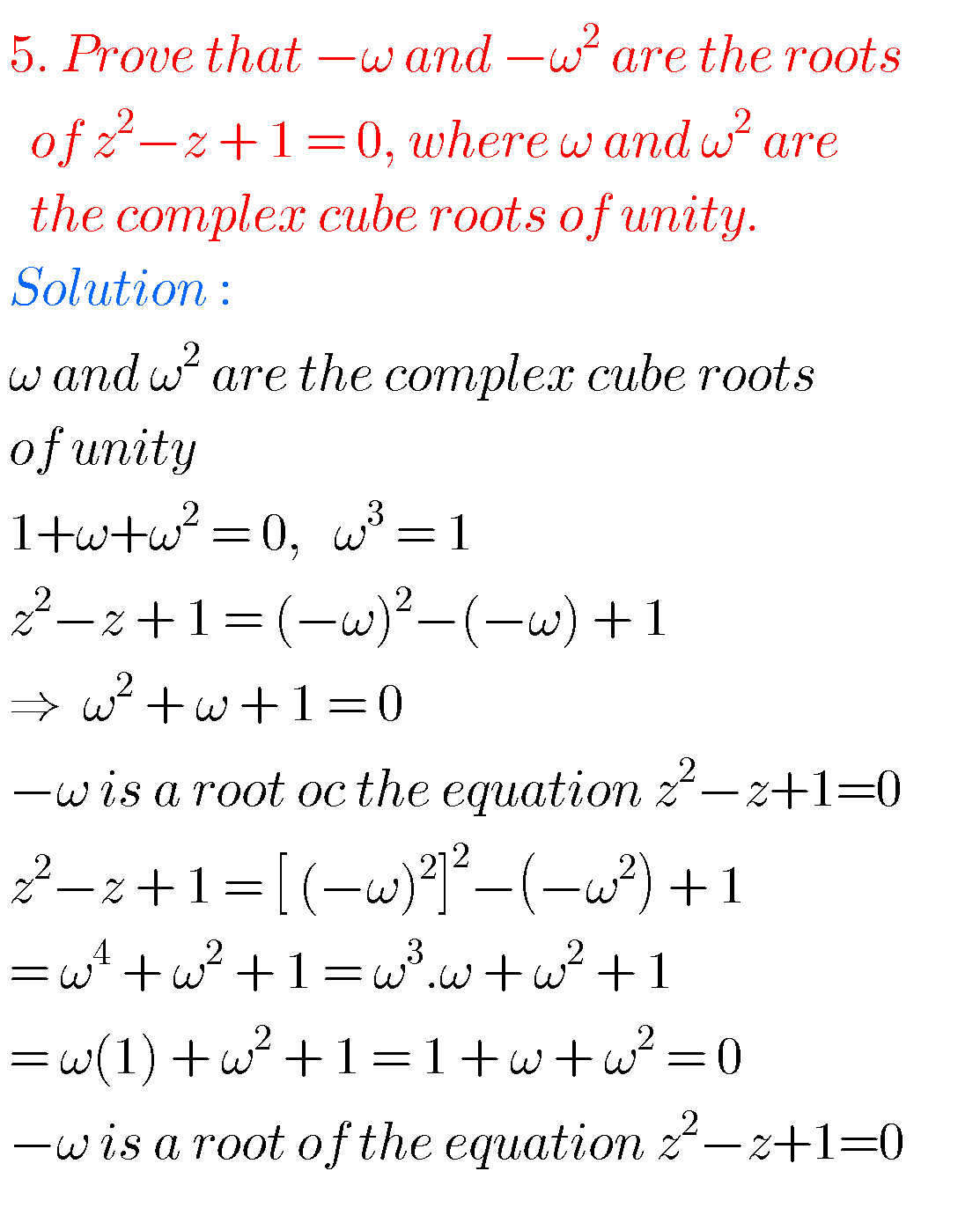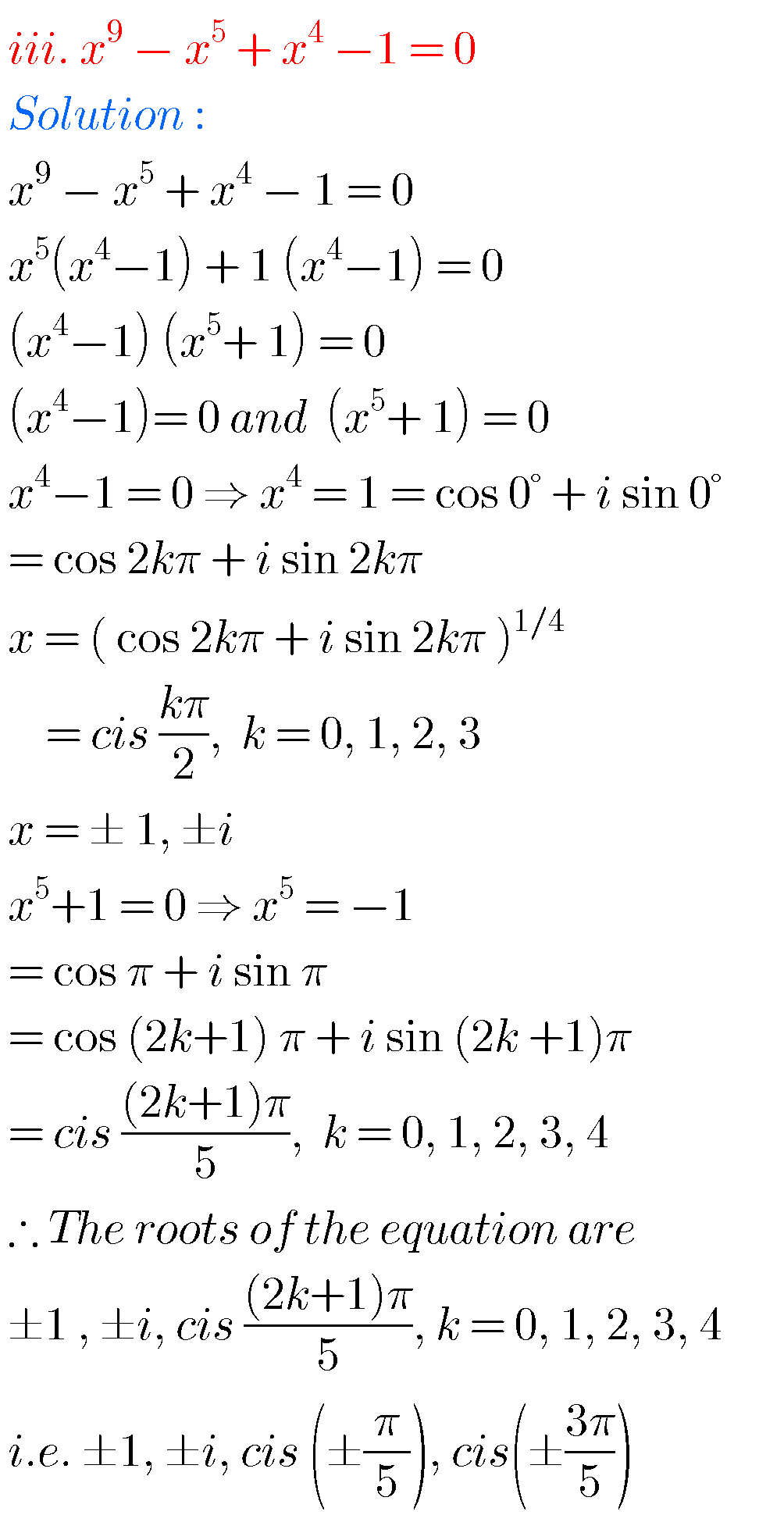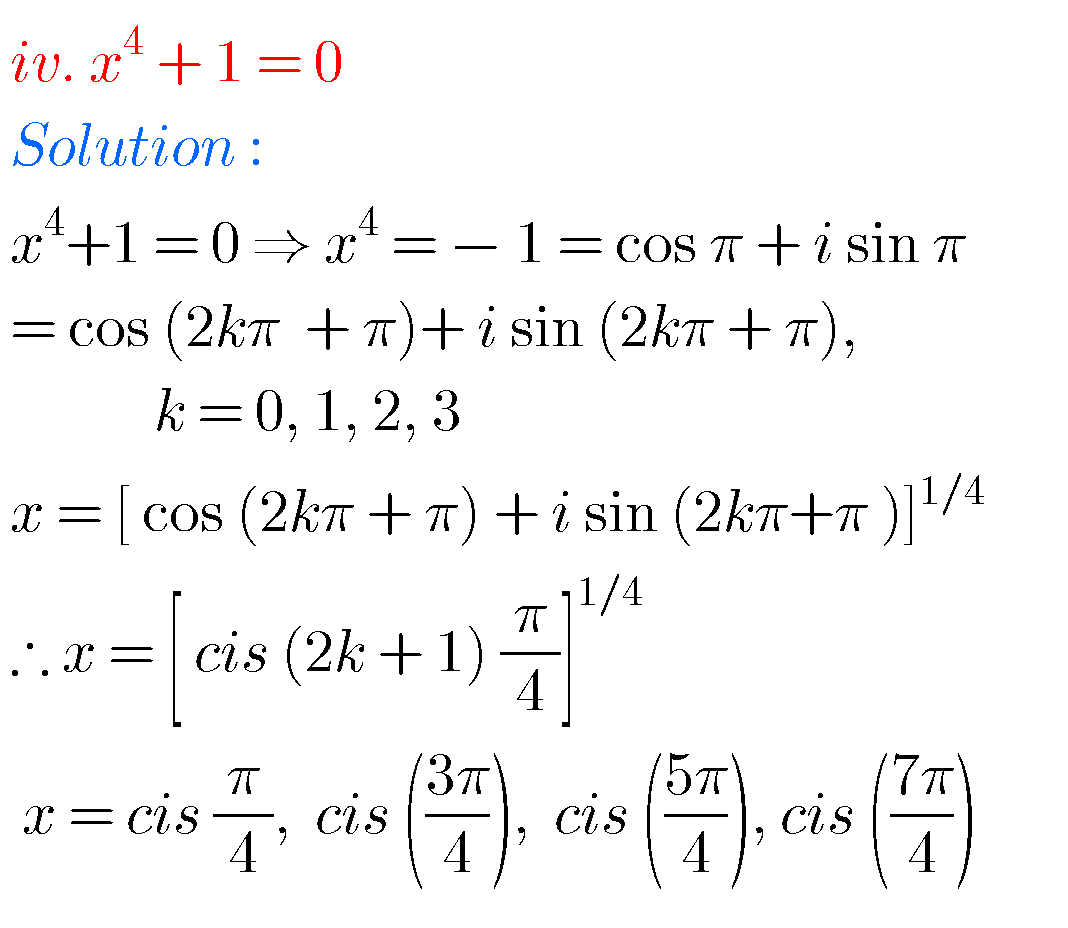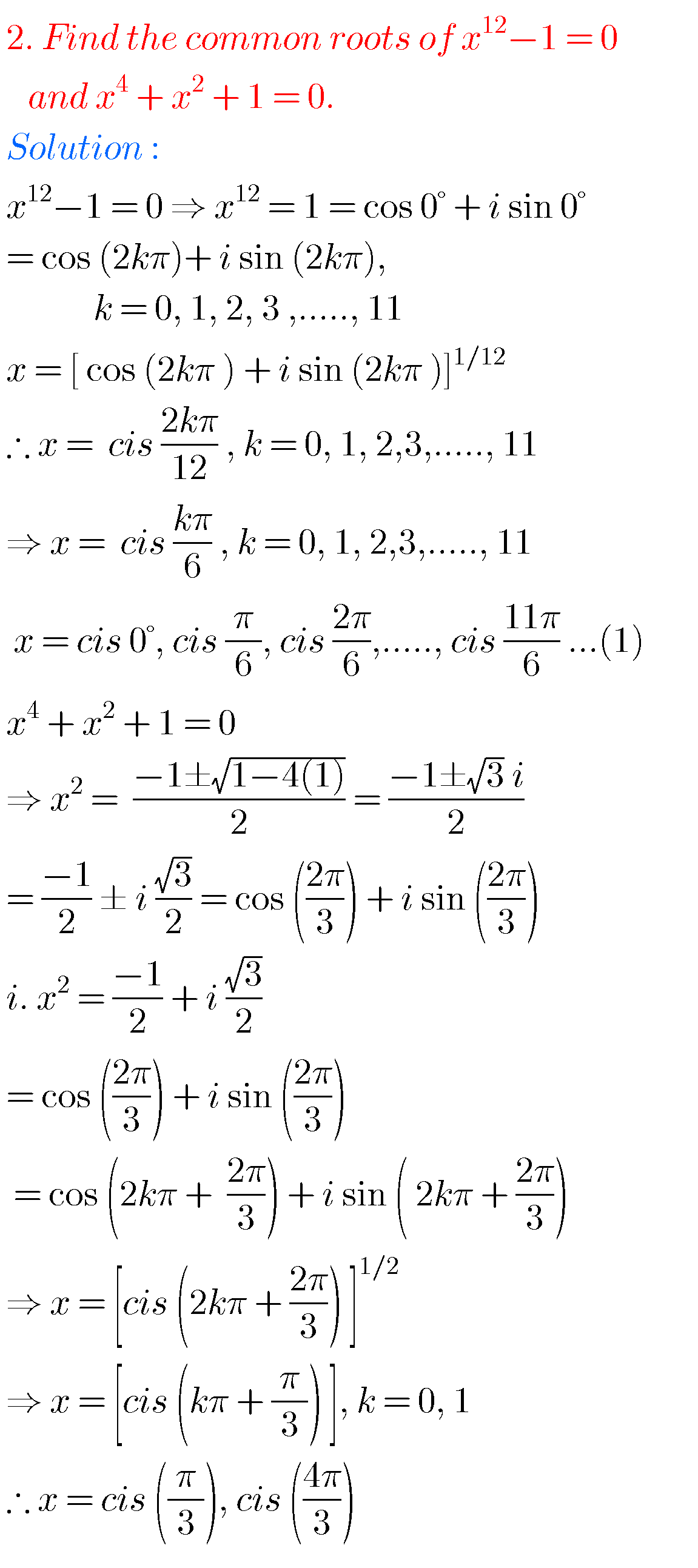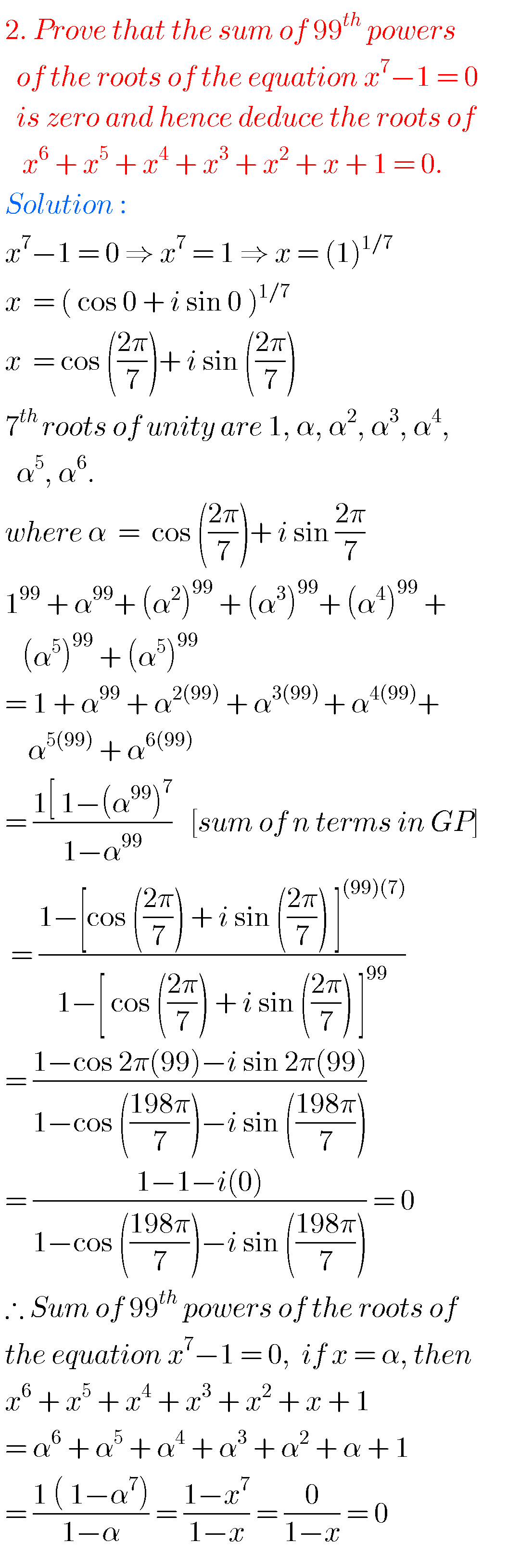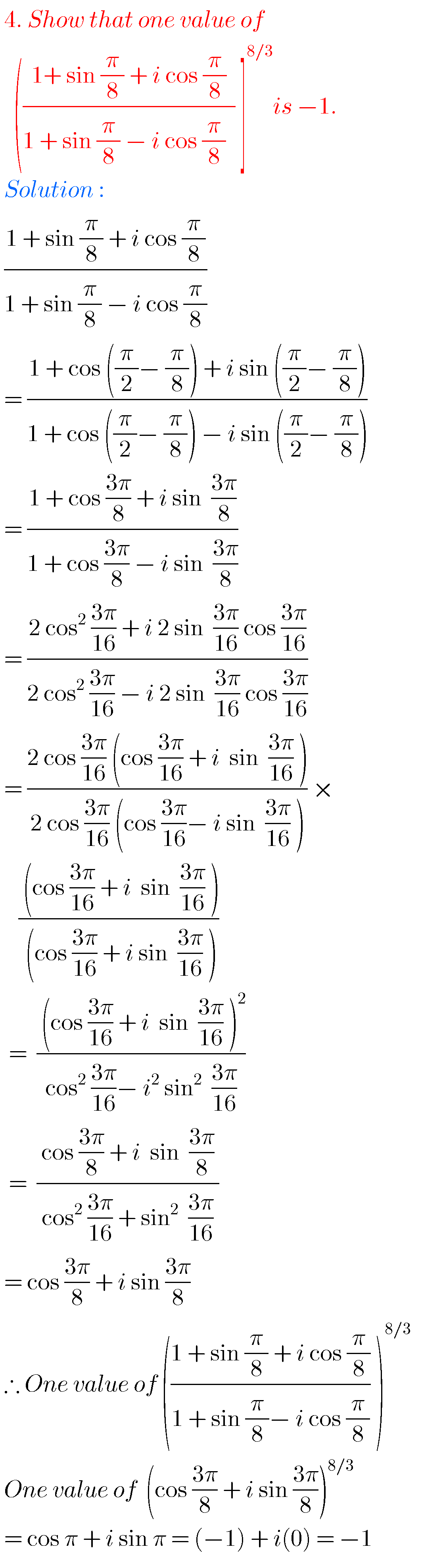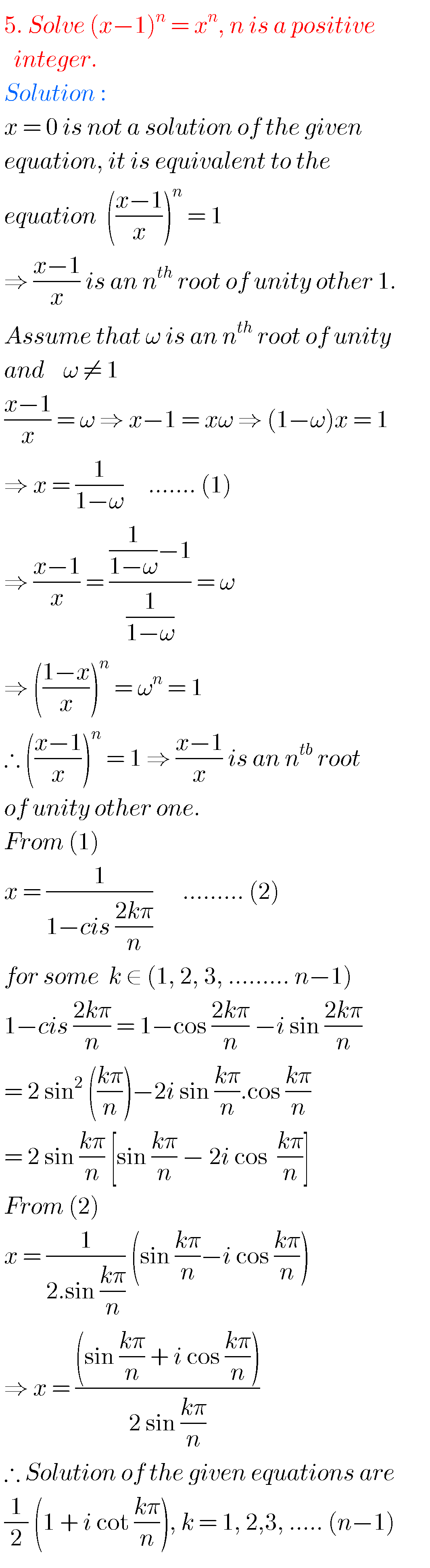Note : Observe the solutions and try them in your own method.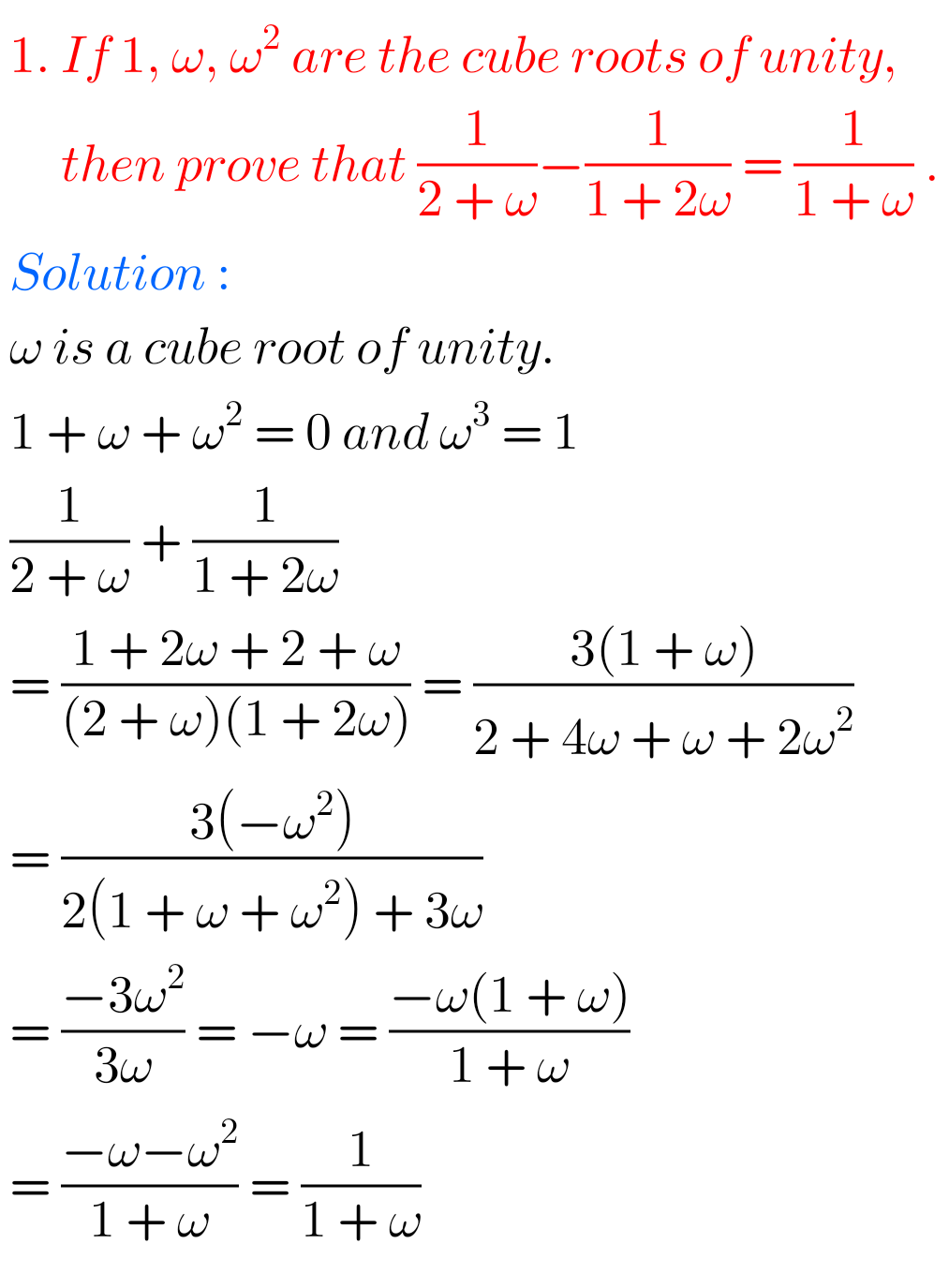Some more solutions

Inter 1a exercise 6(a) solutions

Inter maths 1a exercise 6(b) solutions

class 10 maths polynomials solutions

Ncert maths class 8 exponents and powers

Ncert maths class 7 fractions and decimals

Ncert maths class 6 playing with numbers

Ncert solutions for maths class 7 rational numbers

Ncert solutions for maths class 6 knowing our numbers

Ncert solutions for maths class 6 integers

Ncert solutions for maths class 7 integers

Ncert solutions for maths class 6 whole numbers